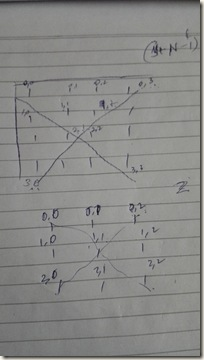## Friday, 10 July 2015

### Diagonal read the matrix array - C++

Introduction

After a long time, here comes a post on parsing a NxN matrix. Our main intension here is to take the sum of the diagonals and take a absolute difference between them. At first, I simply forgot the C++ way to initialize an NxN array. Whoof!!

Let’s first understand what a double pointer is. It’s just a pointer to an array of pointers!!

That is,

What we do with a integer pointer *A? . Allocate an array of integers

Then what to do when u need a list of integer pointers **A ?  Allocate an array of integer pointers

How its done?

Now below is the code to allocate the double pointer

`int N=10;`
`int **A = new int*[N]; // get array of N integer pointers`
`for(int i = 0; i < N; i++)`
`    A[i] = new int[N]  // now allocate to each pointer an array of N`
` `
`// finally A[i][j] gets you into NxN!!`

Next confusion comes with reading this array diagonally. Until we understand how coordinates of an diagonal behave, it’s tough to understand that clearly.If you see the left diagram, you can guess the paper work done for it.

From left->right, you can notice that always you get two indices, (i == j) equal. So you got this diagonal for all NxN.

From right –> left, what is the pattern ? It has to be the combination of i and j. If you see closely, i increases and j decreases as we move. i always increases in our loop, we just need to take care of making j to decrease.. or equating like this as well  i+j = N+1 works!!

`#include <cmath>`
`#include <cstdio>`
`#include <vector>`
`#include <iostream>`
`#include <algorithm>`
`using namespace std;`
` `
` `
`int main() {`
`    int N=1;`
`    cin>>N;`
`    int **A = new int*[N];`
`    for(int i = 0; i < N; ++i)`
`    {`
`        A[i] = new int[N];`
`        for(int j = 0; j < N; ++j)`
`        {`
`            cin>>A[i][j];`
`        }`
`    }`
`    `
`    int sumDiag1 = 0;`
`    int sumDiag2 = 0;`
`    for(int i = 0; i < N; ++i)`
`    {`
`        for(int j = 0; j < N; ++j)`
`        {`
`            if(i == j)`
`            {`
`               sumDiag1 += A[i][j];    `
`            }`
`            `
`            if(j + i == N-1)`
`            {`
`               sumDiag2 += A[i][j]; `
`            }`
`        }`
`    }`
`    cout<<abs(sumDiag1 - sumDiag2);`
`    return 0;`
`}`

DISCLAIMER: There are better ways to do this on internet without O(N^2) loop. You can do this in a single loop as well.